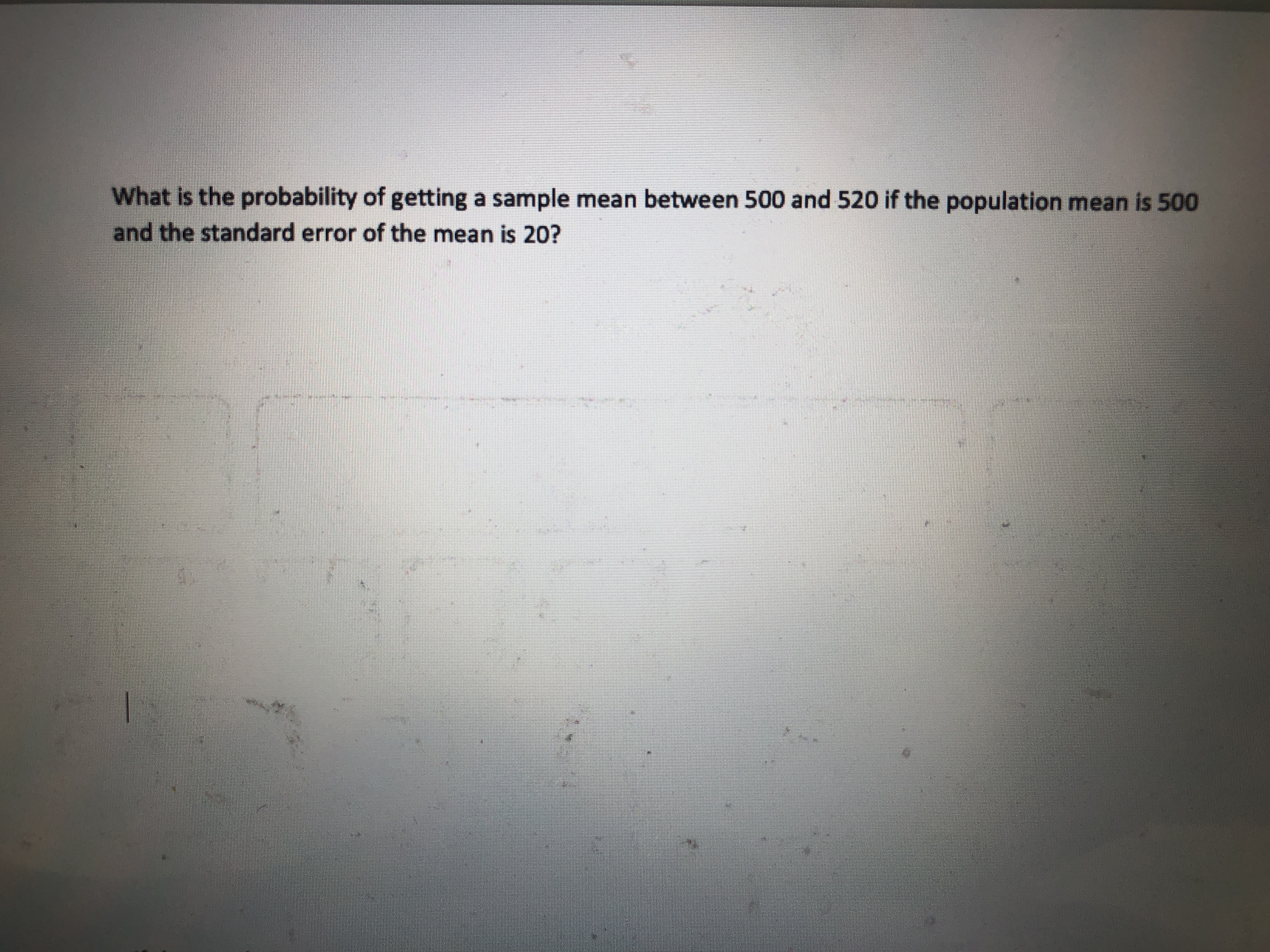# What is the probability of getting a sample mean between 500 and 520 if the population mean is 500and the standard error of the mean is 20?

Question
2 viewshelp_outlineImage TranscriptioncloseWhat is the probability of getting a sample mean between 500 and 520 if the population mean is 500 and the standard error of the mean is 20? fullscreen
check_circle

Step 1

The population mean is 500 and the standard error of the mean is 20.

The probability of ge...

### Want to see the full answer?

See Solution

#### Want to see this answer and more?

Solutions are written by subject experts who are available 24/7. Questions are typically answered within 1 hour.*

See Solution
*Response times may vary by subject and question.
Tagged in
MathStatistics

### Other